# Electric Current Electric Current I Amount of charge

• Slides: 19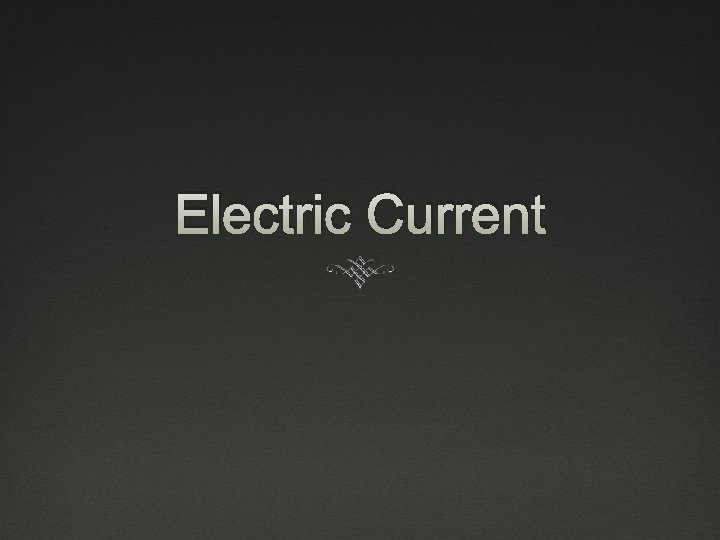Electric CurrentElectric Current (I) Amount of charge transferred through an area per second I = q/T I = current (A, amperes) q = charge (C, coulombs) T = time (seconds, s)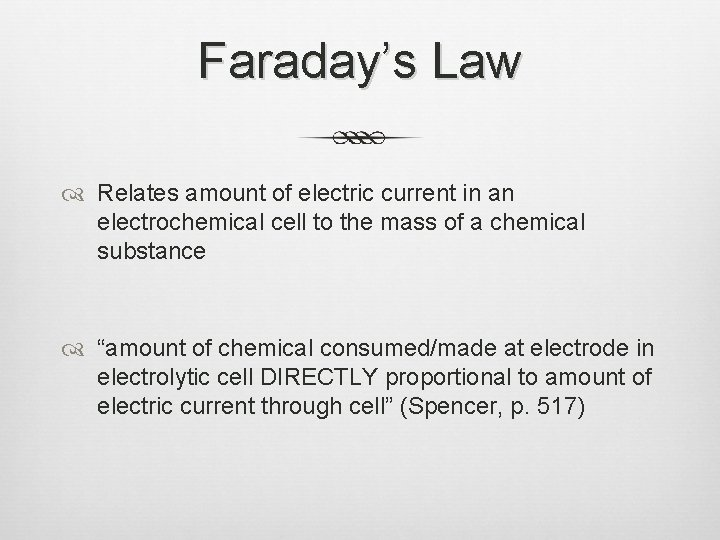Faraday’s Law Relates amount of electric current in an electrochemical cell to the mass of a chemical substance “amount of chemical consumed/made at electrode in electrolytic cell DIRECTLY proportional to amount of electric current through cell” (Spencer, p. 517)Faraday’s constant (F) States the amount of charge (C) transferred by one mole of electrons F = 96, 500 C/mol of electrons Stoichiometric relationship between mole of electrons and mole of chemical substance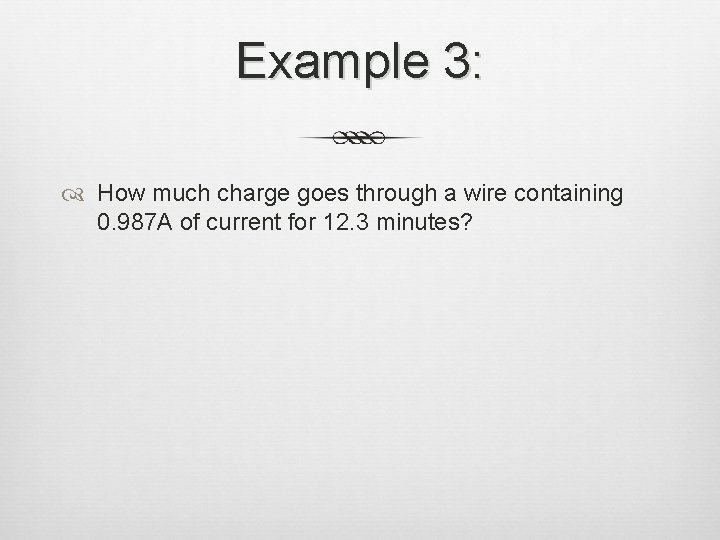Example 3: How much charge goes through a wire containing 0. 987 A of current for 12. 3 minutes?Example 4: How many moles of electrons went through the area in Example 3?Example 5: Calculate the volume of H 2 gas at 25°C and 1 atm that will be produced at the cathode when water undergoes electrolysis for 2. 0 hours at a 10. 0 A electric current.Electrolytic Cells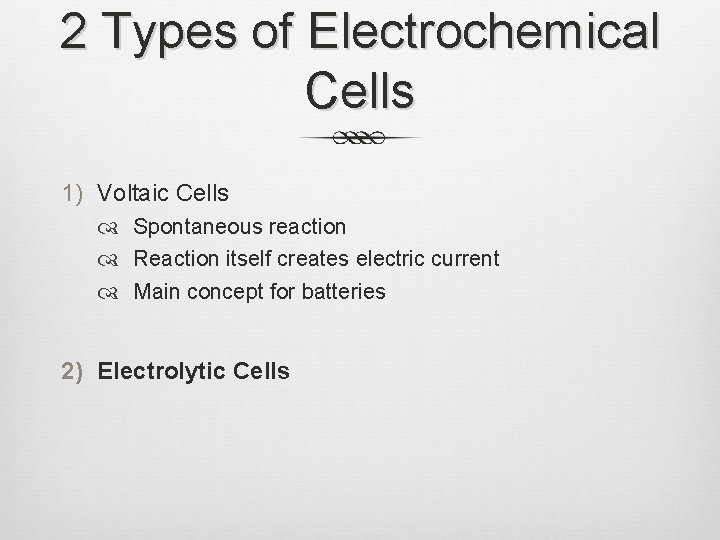2 Types of Electrochemical Cells 1) Voltaic Cells Spontaneous reaction Reaction itself creates electric current Main concept for batteries 2) Electrolytic CellsElectrolytic Cells Reaction is NONSPONTANEOUS Electric current drives redox reaction External source for electrical current—serves as “electron pump” Electrolysis Process of using an electric current to drive chemical reaction Ex. ElectroplatingElectrolytic Cells (cont. ) Electrodes submerged into a salt solution, NO salt bridge Anode Picks up electrons from solution, negative ions give up electrons Oxidation still occurs here Cathode Receives electrons from anode/wire Positive ions accept electrons Reduction still occurs here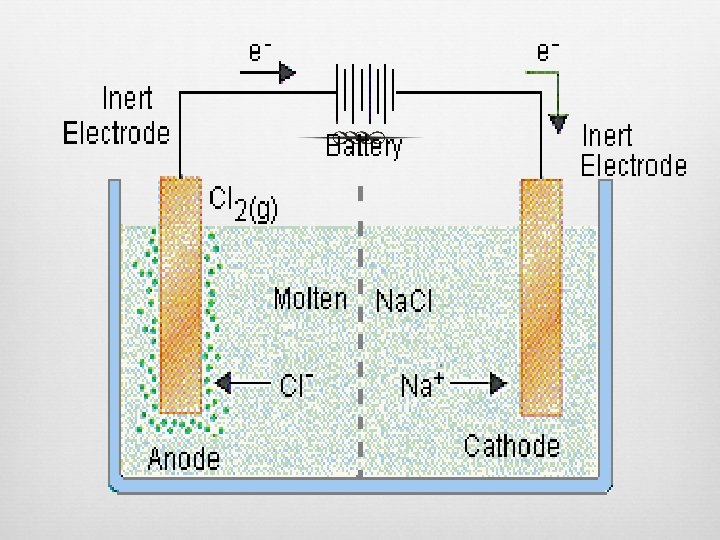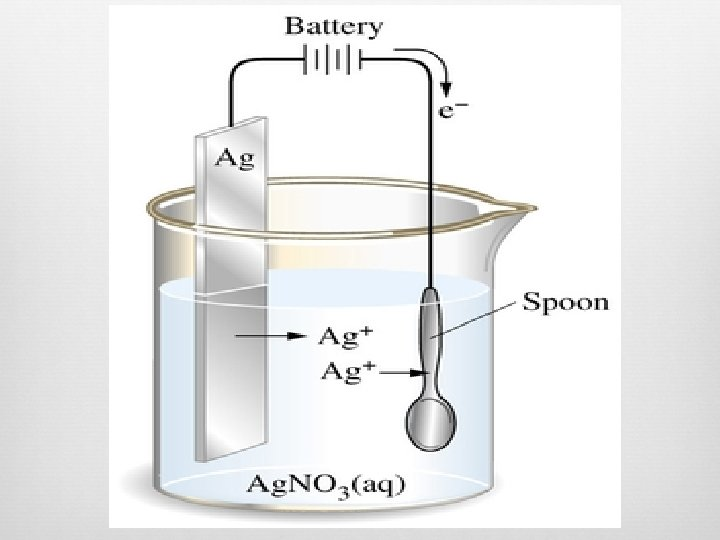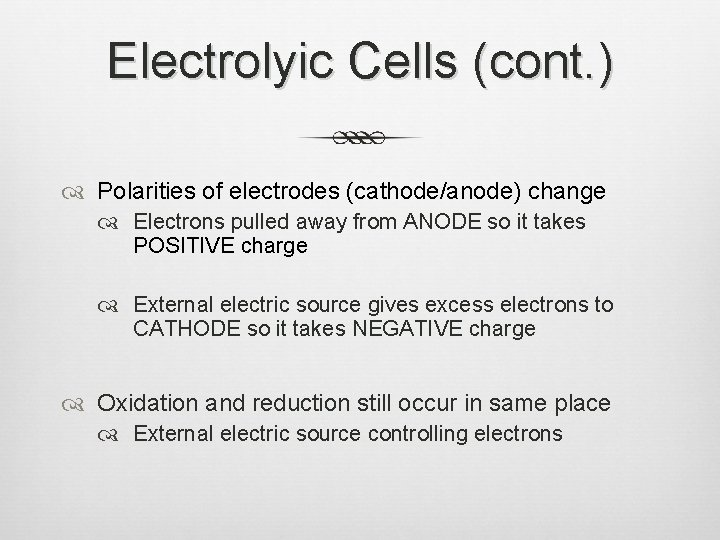Electrolyic Cells (cont. ) Polarities of electrodes (cathode/anode) change Electrons pulled away from ANODE so it takes POSITIVE charge External electric source gives excess electrons to CATHODE so it takes NEGATIVE charge Oxidation and reduction still occur in same place External electric source controlling electrons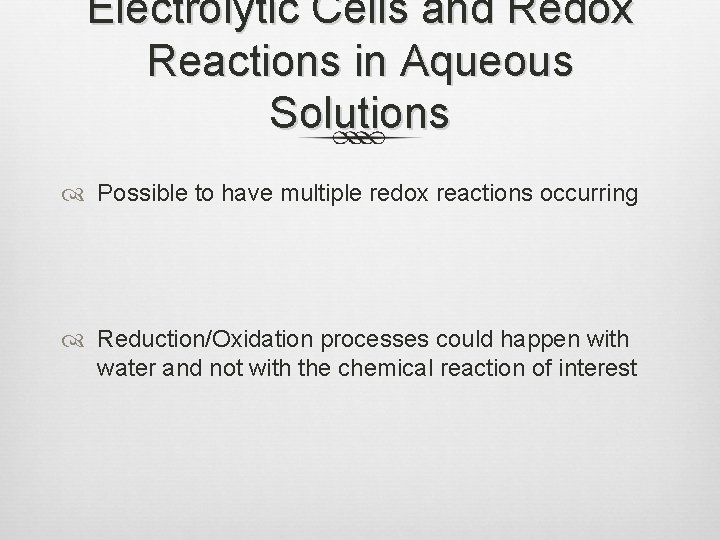Electrolytic Cells and Redox Reactions in Aqueous Solutions Possible to have multiple redox reactions occurring Reduction/Oxidation processes could happen with water and not with the chemical reaction of interest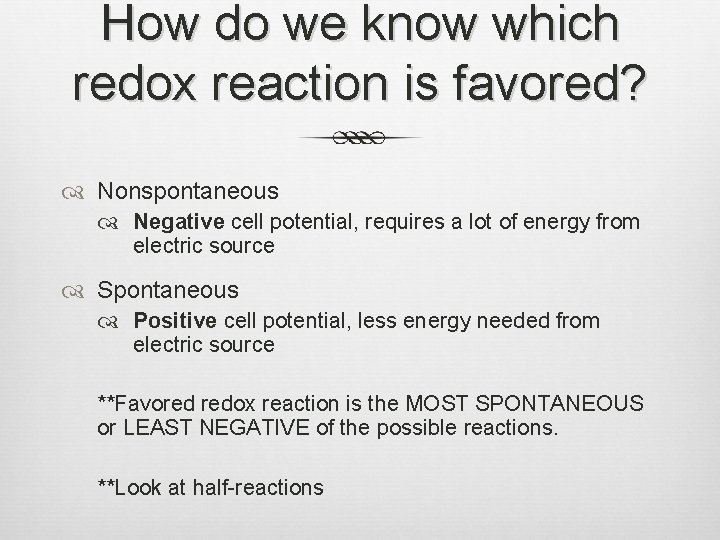How do we know which redox reaction is favored? Nonspontaneous Negative cell potential, requires a lot of energy from electric source Spontaneous Positive cell potential, less energy needed from electric source **Favored redox reaction is the MOST SPONTANEOUS or LEAST NEGATIVE of the possible reactions. **Look at half-reactionsExample 1: Electrolysis of Ca. I 2 Reduction: Ca+2 + 2 e- Ca(s) Ered = -2. 87 V 2 H 2 O + 2 e- H 2 + 2 OH- Ered = -0. 83 V Oxidation: 2 I- I 2 + 2 e- Ered = +0. 53 V 2 H 2 O O 2 + 4 H+ + 4 e- Ered = + 1. 23 V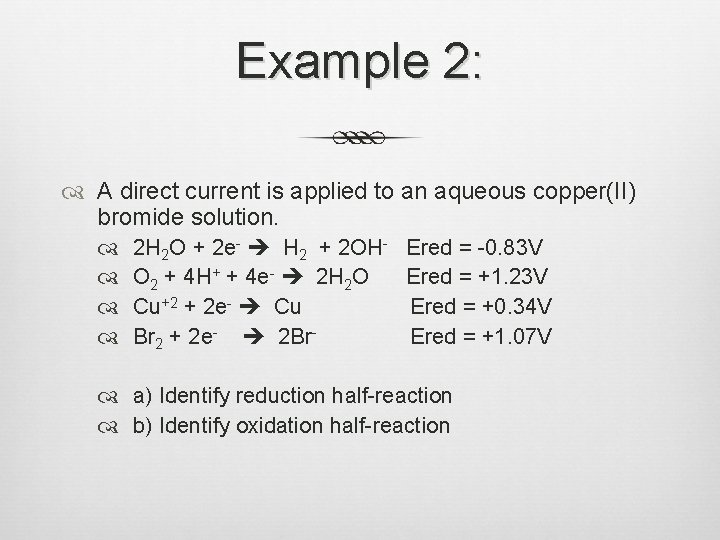Example 2: A direct current is applied to an aqueous copper(II) bromide solution. 2 H 2 O + 2 e- H 2 + 2 OH- Ered = -0. 83 V O 2 + 4 H+ + 4 e- 2 H 2 O Ered = +1. 23 V Cu+2 + 2 e- Cu Ered = +0. 34 V Br 2 + 2 e- 2 Br. Ered = +1. 07 V a) Identify reduction half-reaction b) Identify oxidation half-reaction